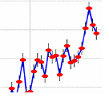# My Climate Change News

 Climate Facts

## Royal Society Publishing

This Fact Page displays text and images related to global warming and climate change
(Hover your mouse over the text below to "popup" a window with a related text.
Click on the text or image to open a new window with a detailed description.)

 Figure 1.Mechanisms influencing WNV transmission.(a) Variables (blue) that influence human WNND cases (red) either positively (green arrows) or negatively (black arrows), either directly, or via effects on mosquito populations (purple). Note that it is the product of mosquito abundance and prevalence that determines risk to humans. (b–e) The fitted relationships for the temperature-dependent (b) biting rate , (c) mortality rate , and (d) the inverse of the extrinsic incubation period (L.D.K., A. C. Matacchiero, A.T. Ciota & A.M.K. 2013, unpublished data) were used to generate (e) the resulting estimated relationships between temperature and partial-R0 for West Nile virus for C. tarsalis (triangles, dashed lines), C. pipiens (circles, solid lines) and C. quinquefasciatus (cross-hatches, dotted lines; see Material and methods). (Online version in colour.) Figure 2.Climate and immunity correlations with annual state WNND cases.The effect of (a) immunity (cumulative incidence; coeff. = -2.05, F1,300 = 96.42, p < 0.001), (b) precipitation (coeff. = -0.0009, F1,161 = 2.20, p = 0.14), (c) drought (coeff. = -0.14, F1,274 = 27.01, p < 0.001), (d) winter severity (coeff. = -0.05, F1,34 = 2.95, p = 0.09), (e) temperature (PIP: coeff. = 0.06, F1,276 = 2.58, p = 0.10; TAR: coeff. = 0.22, F1,144 = 53.59, p < 0.001; QUI: coeff. = 0.002, F1,104 = 0.0005, p = 0.98) and (f) temperature modelled as the relative R0 value at a given temperature (coeff. = 1.66, F1,121 = 17.33, p < 0.001) on the total logged number of WNND cases (adjusted for state random effects) in a given state and year (1999–2013). In (a–d,f), the filled red points and fitted lines are univariate regressions for states in which that predictor was significant (a < 0.05), while open black points depict states in which the predictor was not significant. In (e), green crosses, blue circles and green triangles denote states where C. tarsalis, C. pipiens and C. quinquefasciatus, respectively, dominate transmission and the relationship is only significant for C. tarsalis. (Online version in colour.) Figure 3.Climate, immunity and WNND cases.(a) Yearly WNND cases and fitted model (line) in nine representative states, and projections of the number of future cases under mean (M) or extreme (E—95th percentile) climate conditions for either current (cross-hatch) or future (star) climate projections. Error bars include both the standard error of the mean projected values and the standard deviation of the residuals between current projected and actual values. (b) Colours/shading indicate the significant variables in the fitted models by state with pie-charts showing their relative importance. (c) Human WNND cases and abundance of infected mosquitoes in Colorado when all humans were naive (2003, filled points, coeff = 0.99, F1,175 = 173.1, p < 0.001) and in subsequent years (2004–2008, open points, coeff = 0.72, F1,787 = 285.5, p < 0.001). Variables were power transformed (1/4) to equalize leverage and linearize the relationship. (Online version in colour.) Figure 4.(a) National historical and projected WNND cases.Interannual variation in human WNND cases (filled circle, solid line) and deaths (open circle, dashed line) in the USA and projections of WNND cases under mean current (MC), extreme current (EC—95th percentile) mean future (MF) or extreme future (EF) conditions. Error bars include both the standard error of the mean projected values and the standard deviation of the residuals between current projected and actual values. (b) Summed totals of current maximum number of yearly cases and projected future cases with and without immunity in states where immunity was (grey) or was not (black) significant.